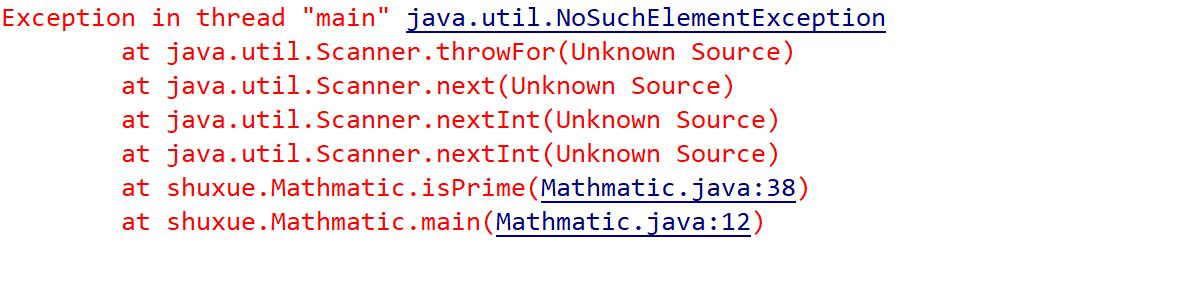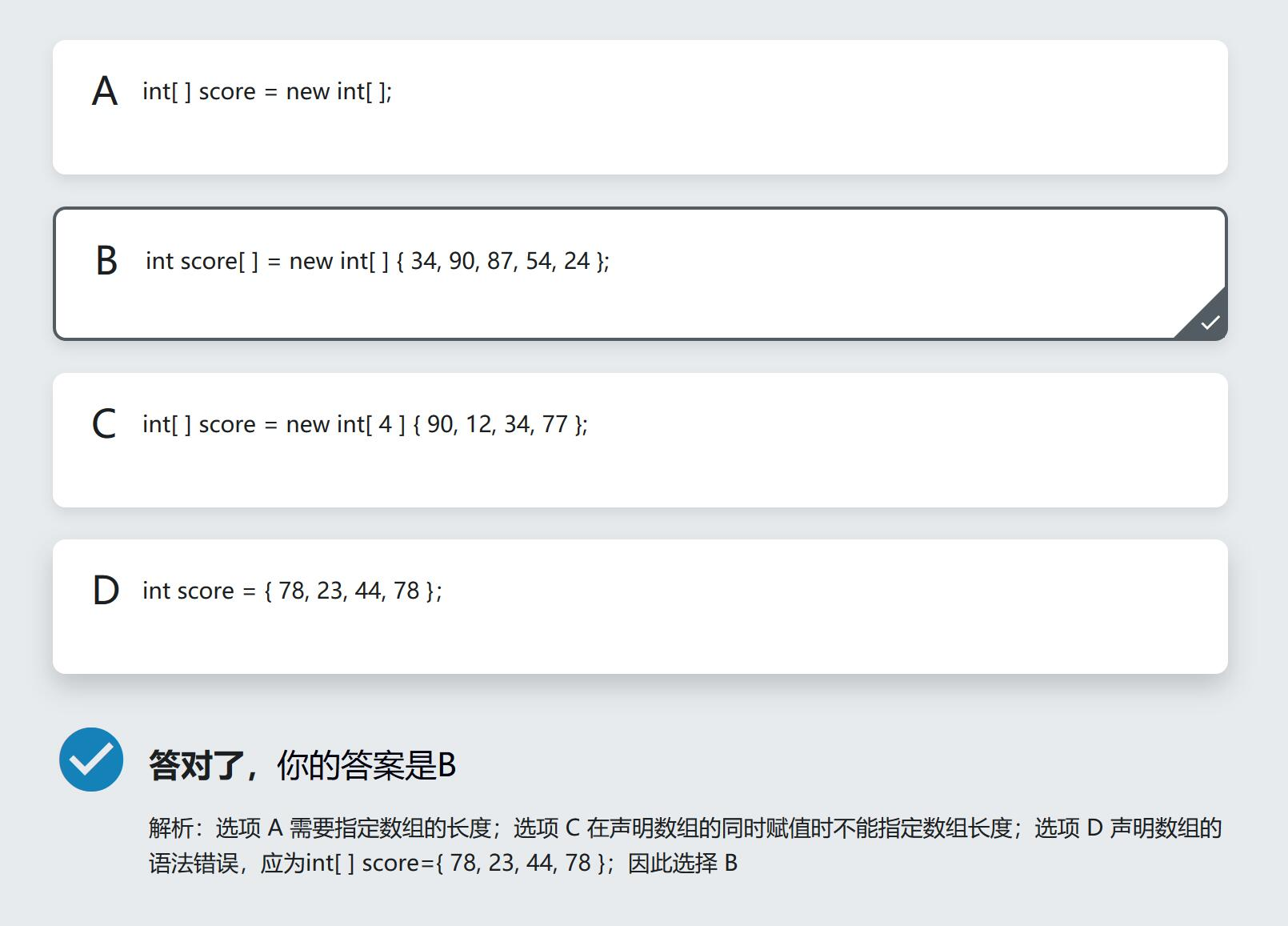# 原代码:

```		for(int i=0;i<tabName.length;i++) {
Btn = new JButton(tabName[i]);
Btn.setPreferredSize(new Dimension(100,25));
@Override
public void actionPerformed(ActionEvent e){
tabObj.setSelectedIndex(i);//BUG
}
});
}
```

# 解决方案:

```		for(int i=0;i<tabName.length;i++) {
Btn = new JButton(tabName[i]);
Btn.setPreferredSize(new Dimension(100,25));
final int j=i;//通过这个转换,把一个无范围的匿名变量转换成final变量 //FIX
@Override
public void actionPerformed(ActionEvent e){
tabObj.setSelectedIndex(j);//FIX
}
});
}
```

# 问题代码:

```import java.util.*;

public class Mathmatic {

public static void main(String[] args) {
Mathmatic math = new Mathmatic();
int result;
result = math.compare();
System.out.println(result);
result = math.isPrime();
System.out.println(result);
}

public int compare() {
int num1,num2;
Scanner input = new Scanner(System.in);
System.out.println("请输入第一个数字:");
num1=input.nextInt();
System.out.println("请输入第二个数字:");
num2=input.nextInt();
input.close();
if(num1==num2) {
return 0;
}else if(num1>num2) {
return 1;
}else if(num1<num2) {
return -1;
}
return 2;
}

public int isPrime() {
int prime;
Scanner input = new Scanner(System.in);
System.out.println("请输入一个数字:");
prime=input.nextInt();
for(int i=2;i<prime;i++) {
if(prime%i==0) {
return 0;
}
}
return 1;
}
}
```# 错误定位:

```		num2=input.nextInt();
-->input.close();<--
```

# 解决方法:

## [转载][笔记]Java 中的 static 使用之静态方法

1、 静态方法中可以直接调用同类中的静态成员，但不能直接调用非静态成员。如：2、 在普通成员方法中，则可以直接访问同类的非静态变量和静态变量，如下所示：3、 静态方法中不能直接调用非静态方法，需要通过对象来访问非静态方法。如：## [笔记]Java声明数组的正确姿势